# Class 10 Maths notes for Coordinate geometry

In this page we will explain the topics for the chapter 7 of Coordinate geometry Class 10 Maths.We have given quality Coordinate geometry Class 10 Notes to explain various things so that students can benefits from it and learn maths in a fun and easy manner, Hope you like them and do not forget to like , social share and comment at the end of the page.
Table of Content

## Introduction

• We require two perpendicular axes to locate a point in the plane. One of them is horizontal and other is Vertical.The plane is called Cartesian plane and axis are called the coordinates axis
• The horizontal axis is called x-axis and Vertical axis is called Y-axis
• The point of intersection of axis is called origin.
• The distance of a point from y axis is called x –coordinate or abscissa and the distance of the point from x –axis is called y – coordinate or Ordinate
• The x-coordinate and y –coordinate of the point in the plane is written as (x, y) for point and is called the coordinates of the point
• Above all in details can be read in Class IX Maths Coordinate Geometry notes

## Distance formula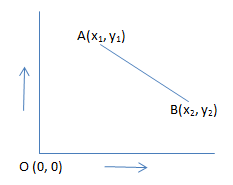Distance between the points AB is given by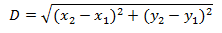Distance of Point A from Origin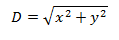(1) Find the distance of point (3,4) and ( 4,3) from Origin?
Solution
Distance from Origin is given by
$D=\sqrt {x^2 + y^2}$
for (3,4),
$D=\sqrt {3^2 + 4^2} =5$
for (4,3),
$D=\sqrt {4^2 + 3^2} =5$

Practice Questions
(1) Arrange these points in ascending order of distance from origin
(a) (1,2)
(b) (5,5)
(c) (-1, 3)
(d) (1/2, 1)
(e) (.5 ,.5)
(f) (-1,1)

(2) Find the distance between the points
(a) (1,2) and (3,4)
(b) (5,6) and ( 1,1)
(c) (9,3) and ( 3,1)

## Section Formula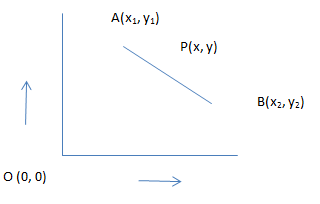A point P(x,y) which divide the line segment AB in the ratio m1 and m1 is given by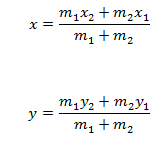The mid point P is given by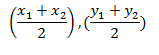## Area of Triangle ABC

Area of triangle ABC of coordinates A(x1,y1) , B(x2,y2) and C(x3,y3) is given by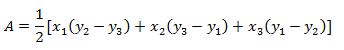For point A,B and C to be collinear, The value of A should be zero

## How to Solve the  line segment bisection ,trisection and four-section problem's

1. You will be given coordinates of the two point A , B
2. If the problem is to find bisection, then you can simply found the mid point using
3. If the problem is to find trisection(three equal parts of the line ).Let us assume the point are P and Q, then AP=PQ=QB
Now P divides the line AB into 1:2 part
While Q divides the line AB into 2:1 part
So we can use section formula to get the coordinate of point P and Q
4. If the problem is to find four equal parts .Let us assume the point are P ,Q And R  such that AP=PQ=QR=RB
Method 1:
Now P divides the line AB into 1:3 part
Q divides the line AB into 1:1 part
R divides the line AB into 3:1 part
So we can use section formula to get the coordinate of point P  ,Q and R
Method 2:
We can find using Mid point also as Q divides A and B in 1:1 and P divides A and Q in 1:1 and R divides Q and B in 1:1

## How to Prove three points are collinear

1) We need to assume that if they are not collinear,they should be able to form  triangle.
We will calculate the area of the triangle,if it comes zero, that no triangle can be found and they are collinear## How to solve general Problems of Area in Coordinate geometry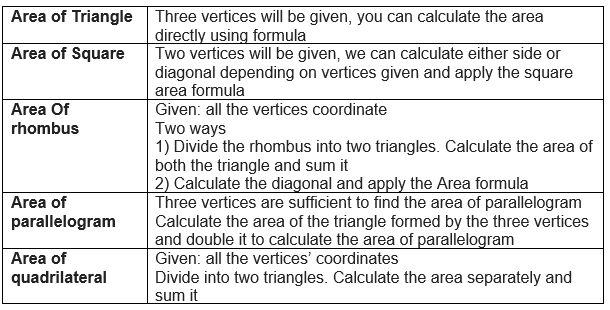## Solved Examples

Question 1
The points (4, 5), (7, 6) and (6, 3) are collinear. True or false
Solution
False. Since the area of the triangle formed by the points is 4 sq. units, the points are not collinear

Question 2
If the mid-point of the line segment joining the points A (3, 4) and B (k, 6) is P (x, y) and
$x + y - 10 = 0$, find the value of k.
Solution
Mid-point of the line segment joining A (3, 4) and B (k, 6) = $\frac {(3+k)}{2}$, 5
Now mid -point is P(x,y)
$x=\frac {(3+k)}{2}$ or 3+k=2x
y=5
Since $x + y - 10 = 0$, we have
$\frac {3+k}{2} +5 - 10 = 0$
3 + k = 10
or k=7

Question 3
ABCD is a parallelogram with vertices A (x1, y1), B (x2, y2) and C (x3, y3). Find the coordinates of the fourth vertex D in terms of x1,x2,x3,y1,y2,y3
Solution
Let the coordinates of D be (x, y). We know that diagonals of a parallelogram bisect each other.
Therefore, mid-point of AC = mid-point of BD
$[ \frac{(x_1 + x_3)}{2} ,\frac{(y_1 + y_3)}{2}]=[ \frac{(x + x_2)}{2} ,\frac {(y + y_2)}{2}]$
i.e $x_1 + x_3 = x_2 + x$ and $y_1 + y_3 = y_2 + y$
$x= x_1 + x_3 - x_2$ and $y=y_1+ y_3 -y_2$
So coordinates are $(x_1 + x_3 - x_2, y_1+ y_3 - y_2)$

Question 4<
The area of a triangle with vertices (a, b + c), (b, c + a) and (c, a + b) is
Solution$A=\frac {1}{2} [a(c+a -a-b) + b(a+b-b-c) + c( b+c -c-a)]$
$A=\frac {1}{2} [ac-bc + ba-bc + bc-ac)]$
A=0

### Quiz Time

Question 1 Point on y axis and x axis has coordinates: ?
A) (a,0) & (0,b)
B) (0,a) & (0,b)
C) (a,b) & (a,b)
D) (0,a) & (b,0)
Question 2 The perimeter of a PQR triangle with vertices P(0, 4), Q(0, 0) and R(3, 0) is: ?
A) 6
B) 7
C) 11
D) 12
Question 3 Which point is at minimum distance from origin
A) (2, -3)
B) (2,2)
C) (6,8)
D) (6,6)
Question 4 The end points of diameter of circle are (0, 0) & (24, 7). The radius of the circle is:
A) 12.5
B) 15.5
C) 12
D) 10
Question 5 The mid point of point A(4,5) and B(-8,7) lies in quadrant?
A) I
B) II
C)III
D) IV
Question 6 The point on the x-axis which is equidistant from (-2,5) and (2, -3) is?
A)(0,3)
B) (0,0)
C)(5,0)
D) (-2,0)

Crossword Puzzle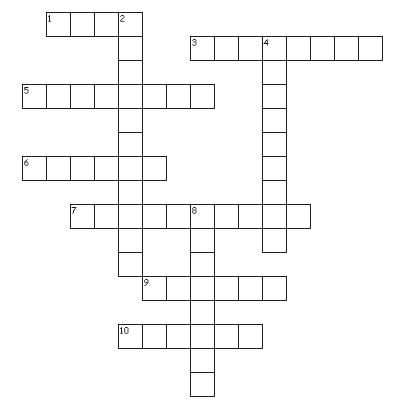Across
1. the distance of point(3,4) from origin
3. x-coordinate is called
5. the y -axis is a .....line
6. The (-6,-2) lies in .....quadrant
7. the x-axis is a ......line
9. The point of intersection of axis
10. The figure formed by joining the point (0,0), (2,0),(2,2) and (0,2)
Down
2. the coordinates (3,0) (-3,0) and $(0,3 \sqrt {3})$ formed an ...... triangle
4. The three point are said to be ....if the area of triangle formed by them is zero
8. y-coordinate is called

(1)five
(2)equilateral
(3)abscissa
(4)collinear
(5)vertical
(6)fourth
(7)horizontal
(8)Ordinate
(9) origin
(10) square

## Summary

Here is Coordinate geometry Class 10 Maths Notes Summary
• The distance between two points is given by $D= \sqrt {(x_2 -x_1)^2 + (y_2 -y_1)^2}$
• The distance of a point from origin is given by $D= \sqrt {x^2 + y^2}$
• The coordinates of the point O(x, y) which divides the line segment joining the points P(x1, y1) and Q(x2, y2) internally in the ratio m : n is given by
$x=\frac {mx_2 + nx_1}{m+n}$ and $y=\frac {my_2 + ny_1}{m+n}$
• The area of the triangle is given by $A= |\frac {1}{2} [x_1(y_2 - y_3) + x_2(y_3 - y-1) + x_3(y_1 -y_2)]|$Go back to Class 10 Main Page using below links

### Practice Question

Question 1 What is $1 - \sqrt {3}$ ?
A) Non terminating repeating
B) Non terminating non repeating
C) Terminating
D) None of the above
Question 2 The volume of the largest right circular cone that can be cut out from a cube of edge 4.2 cm is?
A) 19.4 cm3
B) 12 cm3
C) 78.6 cm3
D) 58.2 cm3
Question 3 The sum of the first three terms of an AP is 33. If the product of the first and the third term exceeds the second term by 29, the AP is ?
A) 2 ,21,11
B) 1,10,19
C) -1 ,8,17
D) 2 ,11,20